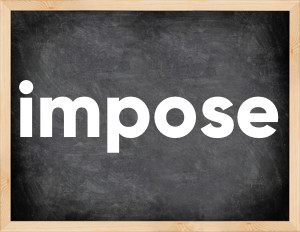# Impose past tenseEnglish verb impose [ɪmˈpəʊz].
Relate to: regular verbs.
3 forms of verb impose: Infinitive (impose), Past Simple - (imposed), Past Participle - (imposed).

## Forms of verb impose in past tenses

👉 Forms of verb impose in future and past simple and past participle.
❓ What is the past tense of impose.

## Three forms of verb impose

Base Form Past Simple Past Participle
impose [ɪmˈpəʊz]

imposed [ɪmˈpəʊzd]

imposed [ɪmˈpəʊzd]

## What is 2nd and 3rd form of impose?

🎓 Base Form (Infinitive) impose in Past Simple, Future Simple, Present Perfect, Past Perfect, Future Perfect?

• First form (V1) - impose. (Present simple, Future Simple)
• Second form (V2) - imposed. (Past simple)
• Third form (V3) - imposed. (Present perfect, Past perfect)

## What are the past tense and past participle of impose?

The past tense of impose is: impose in past simple is imposed. and past participle is imposed.

### What is the past tense of impose?

The past tense of impose is imposed.
The past participle of impose is imposed.

### Verb Tenses

Past simple — impose in past simple imposed. (V2)
Future simple — impose in future simple impose. (will + V1)
Present Perfect — impose in present perfect tense imposed. (have\has + V3)
Past Perfect — impose in past perfect tense imposed. (had + V3)

### impose regular or irregular verb?

👉 Is impose an irregular verb?? impose is regular verb.

## Examples of Verb impose in Sentences

•   Of course we can impose our conditions (Present Simple)
•   Fines are imposed on only 4 persons (Present Simple)
•   Do you know the statement that peace cannot be imposed by force? (Present Simple)
•   They imposed a ten percent tax on tobacco products. (Past Simple)
•   A special tax is imposed on income above average. (Present Simple)
•   A high tax was imposed on alcohol. (Past Simple)
•   The government has imposed onerous taxes on the import of any product. (Present Perfect)
•   Why did the government impose a ban on the sale of ivory? (Past Simple)
•   The government imposed restrictions on the import of foreign cars. (Past Simple)
•   Last year they imposed an economic blockade on this country. (Past Simple)

Along with impose, words are popular flash and dub.

Verbs by letter: , , , , , , , , , , , , , , , , , , , , , , , , .